﻿ Numerical Simulation of the Magnetic Susceptibility of a Spin 1 System Interacting with an Oscillating Magnetic FieldPublications are Open
Access in this journal
Article Versions
Export Article
• Normal Style
• MLA Style
• APA Style
• Chicago Style
Review Article
Open Access Peer-reviewed

### Numerical Simulation of the Magnetic Susceptibility of a Spin 1 System Interacting with an Oscillating Magnetic Field

Applied Mathematics and Physics. 2021, 9(1), 1-4. DOI: 10.12691/amp-9-1-1
Received January 07, 2021; Revised February 08, 2021; Accepted February 22, 2021

### Abstract

We made the numerical simulation of the theoretical results obtained by the resonant means, method in combination with Floquet's theorem of a set of spin particles interacting with an oscillating field. The paces that we obtained by the numerical simulation using Mathematica software of the magnetic susceptibility function theoretically obtained as a function of temperature or as a function of frequency represent a good agreement with the curves of the work published using experimental results. Our objective was to obtain a quality agreement between the experiments and the theoretical model studied.

### 1. Introduction

The study of the magnetic susceptibility of materials has applications in several areas of physics such as electromagnetic, radar application, the optical propagation 1, 3, Photonics 4, 5 and semiconductors 6.

The properties of magnetic susceptibility have aroused the interest of many experimental and theoretical researchers 7. Experimentally observed many properties of themwere good compared to theoretical predictions based on spin theories of fluctuations .Dependence of temperature and frequency of magnetic susceptibility The theory succeeded in deriving the Curie-Weiss law of the magnetic susceptibility of the nonlinear effect of thermal magnetic amplitudes greater than TC 14, 19. It is, however, known that the spontaneous magnetic moment does not vanish within the limit; T → TC 8, 10 20, 21, 22, 23, 24, 25.

In this article, we will show the dependence of the magnetic susceptibility as a function of the frequency for several values of the temperature that we have chosen arbitrarily to compare our theoretical results 11 numerically with the results published in the literature, then we have although showed good agreement with the theoretical and experimental results of several authors published on the same subject 26, 28.

### 2. Theoretical Study

2.1. Floquet Theorem

Consider a quantum system interacting with an oscillating field, Periodical période périodeand amplitude ω1. The Schrödinger equation governing the evolution of this system is such that(1)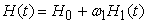(2)

where H0 is the Hamiltonian of the free system , and where H1 is the Hamiltonian which reflects the interaction with aperiodic field. Let V (t) the evolution operator on Eq. (1) V (t) satisfies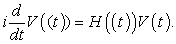(3)

According Floquet 's theorem, there is a decomposition (R, T (t)) such that :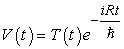(4)

where T (t) is a unitary operator, τ Periodical period (or a multiple de τ), and where R is a constant Hermitian operator.

V (t) is the interaction representation

2.2. Resonating Main Values Method (MMR)

The method of resonant medium (MMR) is due to G. and M. Lochak Thiounn 12 and is a generalization of the method of Bogolyubov 13, 28, 29. It allows the approximate solution of the Schrödinger equation governing the evolution of a quantum system interacting with a periodic field.

The solutions are obtained in the form of a series expansion in powers ω1.The Hamiltonian HI (t) can be written as :(5)

where (HI)k is a constant set of hermetic operators; Ωk which is a sequence of frequencies, which can extract a suite including the frequency Ωk =0.Suppose that this subsequence contains finite number k of frequencies that can direct Ω0 Ω1 Ωk…., And the other Ωk frequencies, Ωk+1 are neither harmonics And the other Ωk frequencies, Ωk+1 are neither harmonics or harmonic combinations of raw k. Then we can write the interaction Hamiltonian in the form:(6)

We define two operators called "middle part" and oscillating part "of (HI)k respectively denotedand asking(7)(8)
2.3. Application of the Method to a Set of Spin One Interacting with an Oscillating Field

Consider a statistical ensemble of N particles with spin 1 without mutual interactions; individual magnetic moment; subjected to a static field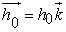and an oscillating field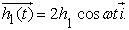The resulting Hamiltonian interaction with these fields is given by:(10)

2.3.1. Susceptibility to Improved First Order

From this result we note that the component of the magnetization depends only on the first order of the intensity of the oscillating field.

Knowing that the magnetic susceptibility can be written: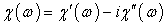(11)

we can deduce the value of the magnetic susceptibility at first order improved depending on the density of the oscillating field , we obtained the following results

The term dispersion is given by the equation: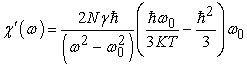(12)

The term absorption is written(13)

Susceptibility to order a is expressed by the equation :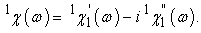(14)

Hence we have deduced the following equation: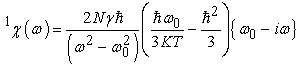(15)

We note that the magnetic susceptibility to order one is linear, and takes its minimum value if ω tends to zero.

2.3.2. Magnetic Susceptibilityto Third Order

In order three , only the Magnetic susceptibility to this order of approximation is given by(16)

We obtained the coefficient of dispersion to order three, it is as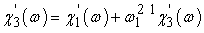(17)

with(18)

We deduced the absorption coefficient at this order of approximation as(19)

or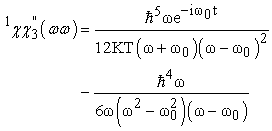(20)

This result highlights the nonlinearity which appeared in the formula of magnetic susceptibility in this order approximation, this translates that has a population of difference in the transitions between the stationary states.

### 3. Simulation

3.1. Variation of Magnetic Susceptibility as a Function of Frequency

The magnetic susceptibility varies linearly as a function of the frequency, and presents a slope which differs for precise values of the temperature.The simulation of the magnetic susceptibility in absorption therm of the following equationas a function of the temperature shows an interesting decrease as the temperature increases. We compared this curve with the one published in the article 11 we will find a good agreement.

• Figure 1. Variation of magnetic susceptibility as a function of ω and T
• Figure 2. Temperature dependence of magnetic susceptibility
• Figure 3. A variation of the dispersion term of order 3 depending on the temperature

### 4. Conclusion

In conclusion, the linear effect is due to the transition level of the photon. In addition, the increase in relaxation time, may improve this effect ; we have also shown that the nonlinearity depends on the temperature , and other parameters such as the structure of the material. We approached the study of a simple example where it is possible, without too many complications, pushing the calculations in higher orders. We have set thus identified "non-linear" interesting effects: saturation effects and nonlinear susceptibility

### References

  A.H. Zaki, M.A. Hafiez, W.M. El Rouby, S.I. El-Dek, A.A. Farghali, Novel magnetic standpoints in Na2Ti3O7 nanotubes, J. Magn. Magn Mater. 476 (2019) 207-212. In article View Article  R.M. Francisco, J.P. Santos, Magnetic properties of the Ashkin-Teller model on a hexagonal nanotube, Phys. Lett. A 383 (11) (2019) 1092-1098. In article View Article  Z. Zhang, Z. Li, J. Zhang, H. Bian, T. Wang, J. Gao, J. Li, Structural and magnetic properties of porous FexOy nanosheets and nanotubes fabricated by electrospinning, Ceram. Int. 45 (1) (2019) 457-461. In article View Article  P. Robkhob, I.M. Tang, S. Thongmee, Magnetic properties of the dilute magnetic semiconductor Zn 1-x Co x O nanoparticles, J. Supercond. Nov. Magnetism (2019) 1-9. In article View Article  A. Gorczyca-Goraj, T. Domanski, M.M. Maska, Topological superconductivity at finite temperatures in proximitized magnetic nanowires, Phys. Rev. B 99 (23) (2019) 235430. In article View Article  Y. Wang, D. Hu, H. Jia, Q. Wang, Efficient enhancement of light trapping in the double-textured Al doped ZnO films with nanorod and crater structures, Phys. B Condens. Matter 565 (2019) 9-13. In article View Article  N.Y. Schmidt, S. Laureti, F. Radu, H. Ryll, C. Luo, F. d’Acapito, M. Albrecht, Structural and magnetic properties of FePt-Tb alloy thin films, Phys. Rev. B 100 (6) (2019), 064428. In article View Article  R. Das, J.A. Cardarelli, M.H. Phan, H. Srikanth, Magnetically tunable iron oxide nanotubes for multifunctional biomedical applications, J. Alloys Compd. 789 (2019) 323-329. In article View Article  A.L. Elrefai, T. Yoshida, K. Enpuku, Magnetic parameters evaluation of magnetic nanoparticles for use in biomedical applications, J. Magn. Magn Mater. 474 (2019) 522-527. In article View Article  N. Lowa, € J.M. Fabert, D. Gutkelch, H. Paysen, O. Kosch, F. Wiekhorst, 3D-printing of novel magnetic composites based on magnetic nanoparticles and photopolymers, J. Magn. Magn Mater. 469 (2019) 456-460. In article View Article  C. Qotni, A. L. Marrakchi, S. Sayouri, Y. Achkar, Effets non linéaires dans l’interaction d’un ensemble de particules de spin 1 avec un champ oscillant dans l'expression de l'aimantation et de la susceptibilité magnétique, I.J.I.A.S. ISSN 2028-9324 vol.17 No. 3 Aug. 2016, pp.1050-1061. In article  G. Lochak. C.R.A.S. Série B, A/272. P.1281.1971. In article  A. Erbeia , Résoances magnétiques-Masson, Paris, 1969. In article  F. Sedighi, M. Esmaeili-Zare, A. Sobhani-Nasab, M. Behpour, Synthesis and characterization of CuWO 4 nanoparticle and CuWO 4/NiO nanocomposite using co-precipitation method; application in photodegradation of organic dye in water, J. Mater. Sci. Mater. Electron. 29 (16) (2018) 13737-13745. In article View Article  B.Z. Mi, C.J. Feng, J.G. Luo, D.Z. Hu, Magnetic compensation and critical properties of a mixed spin-(2, 3/2) Heisenberg single-walled nanotube superlattice, Superlattice. Microst. 113 (2018) 524-533. In article View Article  M.D. Hossain, R.A. Mayanovic, R. Sakidja, M. Benamara, R. Wirth, Magnetic properties of core-shell nanoparticles possessing a novel Fe (II)-chromia phase: an experimental and theoretical approach, Nanoscale 10 (4) (2018) 2138-2147. In article View Article  PubMed  D. Lv, W. Jiang, Y. Ma, Z. Gao, F. Wang, Magnetic and thermodynamic properties of a cylindrical ferrimagnetic Ising nanowire with core/shell structure, Phys. E Low-dimens. Syst. Nanostruct. 106 (2019) 101-113. In article View Article  E. Kantar, Ising-type single-segment ferromagnetic nanowire with core/shell: the dependences of the angle, temperature, and geometry, J. Supercond. Nov. Magnetism 31 (2) (2018) 341-346. In article View Article  H. Magoussi, A. Zaim, M. Kerouad, Theoretical investigations of the phase diagrams and the magnetic properties of a random field spin-1 Ising nanotube with core/shell morphology, J. Magn. Magn Mater. 344 (2013) 109-115. In article View Article  A.S. Tehrani, M.A. Kashi, A. Ramazani, A.H. Montazer, Axially adjustable magnetic properties in arrays of multilayered Ni/Cu nanowires with variable segment sizes, Superlattice. Microst. 95 (2016) 38-47. In article View Article  B. Deviren, M. Keskin, Thermal behavior of dynamic magnetizations, hysteresis loop areas and correlations of a cylindrical Ising nanotube in an oscillating magnetic field within the effective-field theory and the Glauber-type stochastic dynamics approach, Phys. Lett. A 376 (10-11) (2012) 1011-1019. In article View Article  Z. Huang, Z. Chen, S. Li, Q. Feng, F. Zhang, Y. Du, Effects of size and surface anisotropy on thermal magnetization and hysteresis in the magnetic clusters, Eur. Phys. J. B-Condens. Matter Complex Syst. 51 (1) (2006) 65-73. In article View Article  Ü. Akıncı, Crystal field dilution in S-1 Blume Capel model: hysteresis behaviors, Phys. Lett. A 380 (14-15) (2016) 1352-1357. In article View Article  N. Si, J.M. Wang, A.B. Guo, F. Zhang, F.G. Zhang, W. Jiang, Study on magnetic and thermodynamic characteristics of core-shell graphene nanoribbon, Phys. E Lowdimens. Syst. Nanostruct. (2019) 113884. In article View Article  X.W. Quan, N. Si, F. Zhang, J. Meng, H.L. Miao, Y.L. Zhang, W. Jiang, Phase diagrams of kekulene-like nanostructure, Phys. E Low-dimens. Syst. Nanostruct. 114 (2019) 113574. In article View Article  T. Kaneyoshi, Effects of random fields in an antiferromagnetic Ising bilayer film, Phys. E Low-dimens. Syst. Nanostruct. 94 (2017) 184-189. In article View Article  R. Honmura, T. Kaneyoshi, Contribution to the new type of effective-field theory of the Ising model, J. Phys. C Solid State Phys. 12 (19) (1979) 3979. In article View Article  R. Honmura, T. Kaneyoshi, Contribution to the new type of effective-field theory of the Ising model, J. Phys. C Solid State Phys. 12 (19) (1979) 3979. In article View Article  T. Kaneyoshi, J.W. Tucker, M. Jacur, Differential operator technique for higher spin problems, Phys. A Stat. Mech. Appl. 186 (3-4) (1992) 495-512. In article View ArticleThis work is licensed under a Creative Commons Attribution 4.0 International License. To view a copy of this license, visit http://creativecommons.org/licenses/by/4.0/